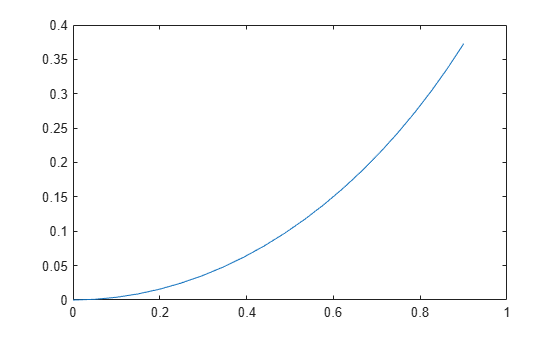# unicycleKinematics

Unicycle vehicle model

## Description

`unicycleKinematics` creates a unicycle vehicle model to simulate simplified car-like vehicle dynamics. The state of the vehicle is defined as a three-element vector, [x y theta], with a global xy-position, specified in meters, and a vehicle heading angle, theta, specified in radians. This model approximates a unicycle vehicle with a given wheel radius, WheelRadius, that can spin in place according to a heading angle, theta. To compute the time derivative states for the model, use the `derivative` function with input commands and the current robot state.## Creation

### Syntax

``kinematicModel = unicycleKinematics``
``kinematicModel = unicycleKinematics(Name,Value)``

### Description

example

````kinematicModel = unicycleKinematics` creates a unicycle kinematic model object with default property values.```
````kinematicModel = unicycleKinematics(Name,Value)` sets additional properties to the specified values. You can specify multiple properties in any order.```

## Properties

expand all

The wheel radius of the vehicle, specified in meters.

The vehicle speed range is a two-element vector that provides the minimum and maximum vehicle speeds, [MinSpeed MaxSpeed], specified in meters per second.

The `VehicleInputs` property specifies the format of the model input commands when using the `derivative` function. Options are specified as one of the following strings:

• `"WheelSpeedHeadingRate"` — Wheel speed and heading angular velocity, specified in radians per second.

• `"VehicleSpeedHeadingRate"` — Vehicle speed and heading angular velocity, specified in radians per second.

## Object Functions

 `derivative` Time derivative of vehicle state

## Examples

collapse all

Create a Robot

Define a robot and set the initial starting position and orientation.

```kinematicModel = unicycleKinematics; initialState = [0 0 0];```

Simulate Robot Motion

Set the timespan of the simulation to 1 s with 0.05 s timesteps and the input commands to 10 m/s and left turn. Simulate the motion of the robot by using the `ode45` solver on the `derivative` function.

```tspan = 0:0.05:1; inputs = [10 1]; %Constant speed and turning left [t,y] = ode45(@(t,y)derivative(kinematicModel,y,inputs),tspan,initialState);```

Plot path

```figure plot(y(:,1),y(:,2))```Lynch, Kevin M., and Frank C. Park. Modern Robotics: Mechanics, Planning, and Control 1st ed. Cambridge, MA: Cambridge University Press, 2017.

##### Support평가판 신청